# Signature

Jump to: navigation, search

The signature of an algebraic system is the collection of relations and operations on the basic set of the given algebraic system together with an indication of their arity. An algebraic system (a universal algebra) with signatureis also called an-system (respectively,-algebra).

The signature of a quadratic, or symmetric bilinear, form over an ordered field is a pair of non-negative integers, whereis the positive andthe negative index of inertia of the given form (see Law of inertia; Quadratic form). Sometimes the number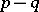is called the signature of the form.

O.A. Ivanova

The signature of a manifold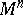is the signature of the quadratic formwhere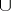is the cohomology cup-product and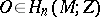is the fundamental class. The manifold is assumed to be compact, orientable and of dimension. The signature is denoted by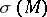.

If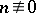, one sets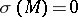. The signature has the following properties:

a);

b)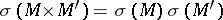;

c)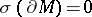.

The signature of a manifold can be represented as a linear function of its Pontryagin numbers (cf. Pontryagin number; ). For the representation of the signature as the index of a differential operator see Index formulas.